# Approach and Practice Questions for Non-verbal Reasoning for SSC exams

Staff Selection Commission (SSC) conducts different types of exams every year. It is the dream for many aspirants to crack the SSC exams. To clear Tier -1 exam in SSC, scoring high is very important. So, aspirants must focus on the non-verbal (visual) reasoning section to maintain a good score as there will be 7-8 questions of non-verbal (visual) reasoning. This article will guide you through the types of Non-verbal Reasoning questions and a few practice questions for the same.

## How to approach Non-verbal Reasoning for SSC:

The Non-verbal Reasoning section in Tier-1 of all types of SSC exams (CGL, CHSL, MTS, Stenographer etc..) carries the weightage of 8-12 marks. Let us give you a complete overview of the approach required to solve Non-verbal Reasoning questions for SSC.

• Relation Between the images/objects in series: The relation between the objects/images in the series should be checked to deduce the logic.
• Applying the Logical Skills: Logical skills play a key role in Non-verbal Reasoning. You should think which logic is applicable to a certain question. These types of problems are solved mostly by applying the logic. Practicing more problems is the best method to improve logical skills.
• Know the concepts: You should learn the concepts of Mirror image, water image, rotation of object/image in anticlockwise/clockwise direction and rotating with an angle etc. These concepts will help you deduce the perfect logic in a very short time.

Attempt a FREE SSC CGL mock test here.

## Types of Non-verbal Reasoning questions for SSC:

### 1. Missing Figure/Finding figure in Series:

In these types of problems, there is a series of figures which are related and form a series with a pattern or logic. The question will be asked to find out the next figure in the series that follows the same logic or the figure that is missing in between the series.

Example:

Select the figure that will come next in the following series.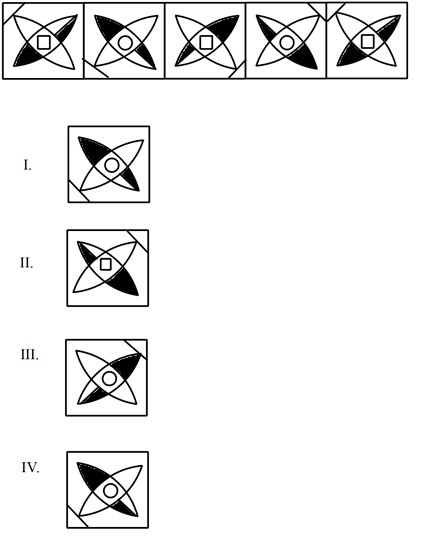(1) I

(2) II

(3) III

(4) IV

Solution:

The line at the corner keeps on rotating in an anticlockwise manner in each step and the remaining figure keeps on rotating in a clockwise manner. In the figure, one part is completely shaded and the other part is partially shaded. The one which is partially shaded changes its shaded side in alternate steps.

The square and circle come in between the figure in alternate steps.

Attempt a FREE SSC CHSL mock here.

### 2. Counting the number of straight lines/Squares/Triangles:

In these types of problems, a figure is given in which you will be asked to find the number of straight lines/triangles/squares/rectangles, etc. For these problems, concentrating and organising the figure in your head is helpful while counting the lines/figures.

Example:

Count the number of triangles in the given figure.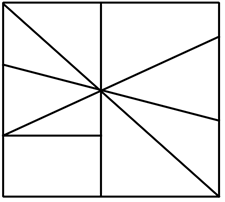(1) 11

(2) 10

(3) 13

(4) 9

Solution: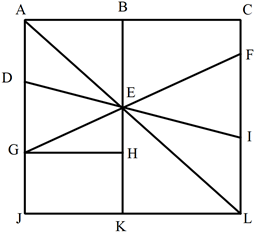ABE, ACL, ADE, AEG, AJL, DEG, EFI, EFL, EGH, EIL, EKL = 11 triangles

Cross out your weak areas by attempting the topic tests on Reasoning.

### 3. Folding the Paper (picture):

In these types of problems, a paper is folded into a small portion and dots or designs are made on it. You will be asked to choose the figure which will be formed when the paper is unfolded.

Example:

Find out from amongst the four alternatives I, II, III and IV, as to how the pattern would appear on the sheet when the sheet is folded at the dotted line and is punched as shown.(1) I

(2) II

(3) III

(4) IV

Solution:

When the paper is opened, we obtain pattern shown in fig II.

Cross out your weak areas by attempting the topic tests on Reasoning.

### 4. Mirror Image/ Water Image:

In these types of problems, the question will be asked to select the image of the picture that will be attained when a mirror is placed on the left/right side of the picture or the water image formed (the image formed on water when the picture is held above water).

Example:

Select the mirror image of the given figure when the mirror is placed to the right of the figure: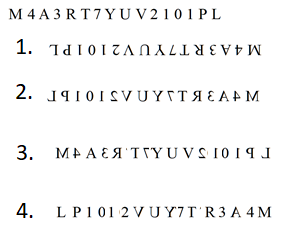(1) 1

(2) 2

(3) 3

(4) 4

Solution:

The image will be as follows: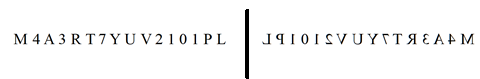### 5. Completing the figure:

A Figure is given such that a part of it will be missing and you will be asked to complete the figure.

Example:

Select the option that completes the given pattern: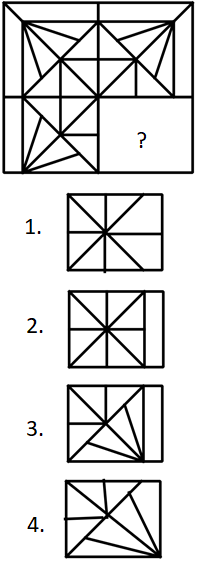(1) 1

(2) 2

(3) 3

(4) 4

Solution:

The complete image will be as follows: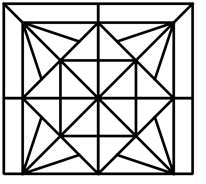Attempt a FREE SSC CGL mock test here.

### 6. Odd One Out:

A series of images is given in which the question is asked to find the odd one out. For these problems, you have to deduce the logic used that makes three/four of the given images similar while makes one stand out among the four/five images.

Example:

In the following question, out of the four figures marked (I), (II), (III) and (IV), three are similar in a certain manner. However, one figure is not like the other three. Choose the figure which is different from the rest.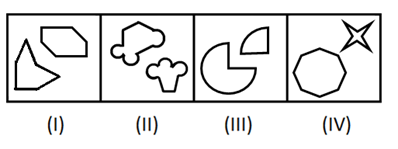(1) I

(2) II

(3) III

(4) IV

Solution:

In each of the figures (I), (III) and (IV), the two shapes have equal number of edges (lines). So, (II) is the odd-one out as one shape has three lines (edges) while the other has four edges.

### 7. Embedded figure:

In these types of problems, a small portion of the figure is given in the question and you will be asked to find the figure from the options in which this small figure is embedded.

Example:

Select the figure in which the given figure is embedded.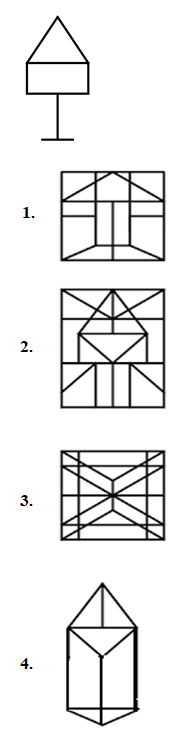(1) 1

(2) 2

(3) 3

(4) 4

Solution:

The complete image will be as follows: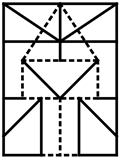We hope this post will help you in visualizing non-verbal reasoning questions for SSC and other exams as well. “Practicing the unknown can make anything known.”